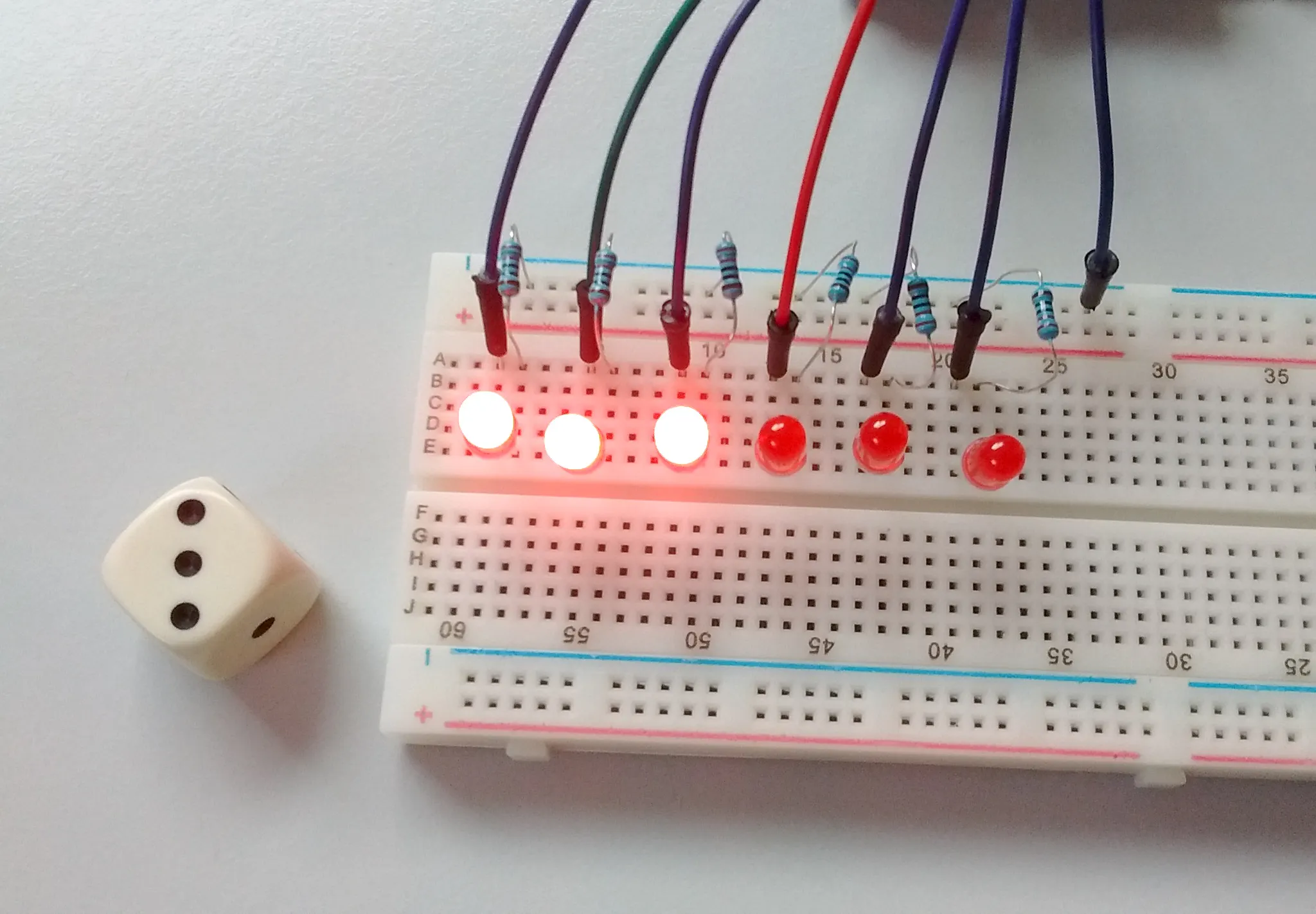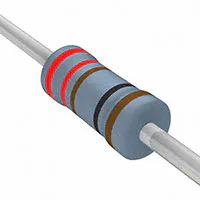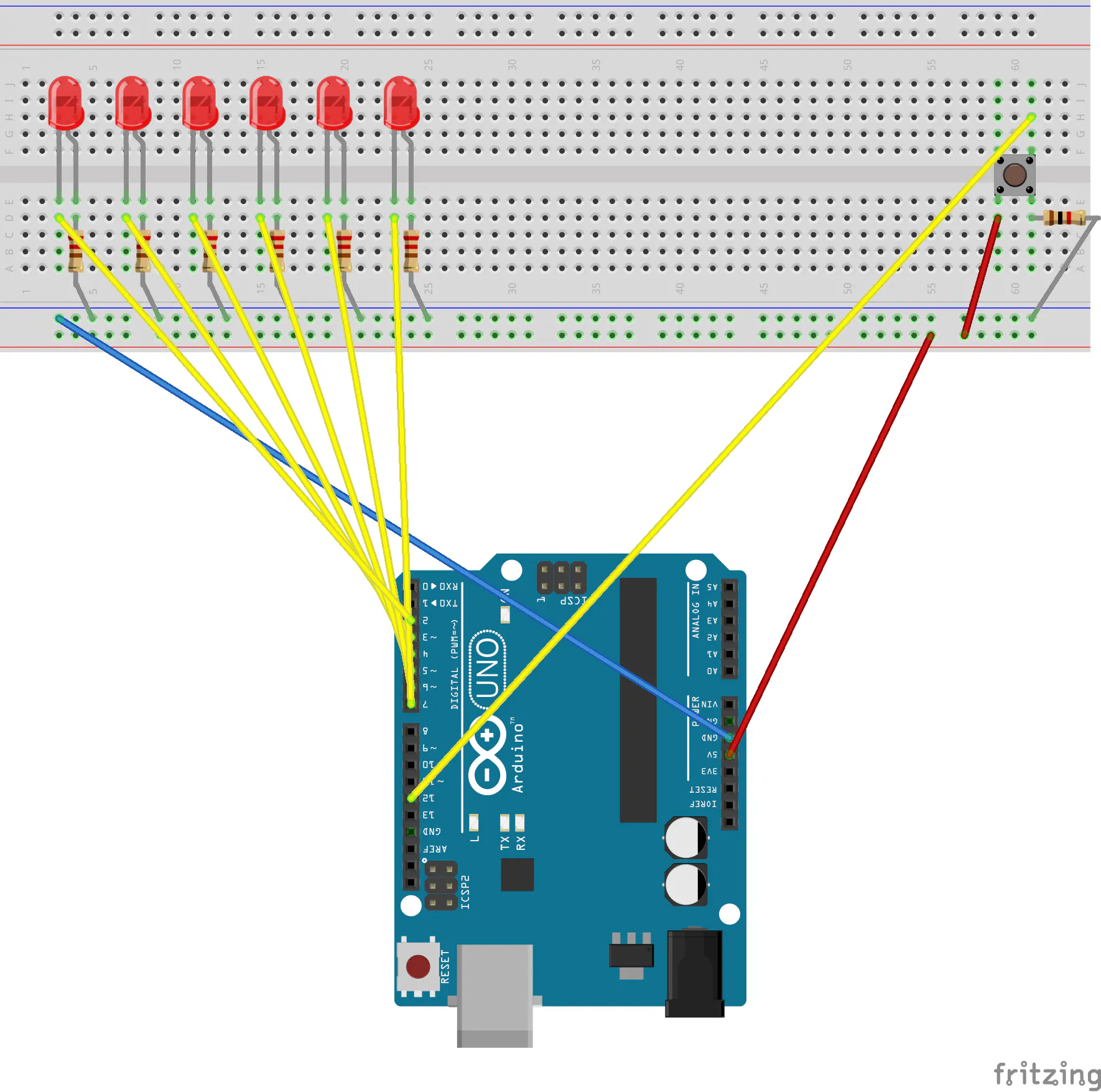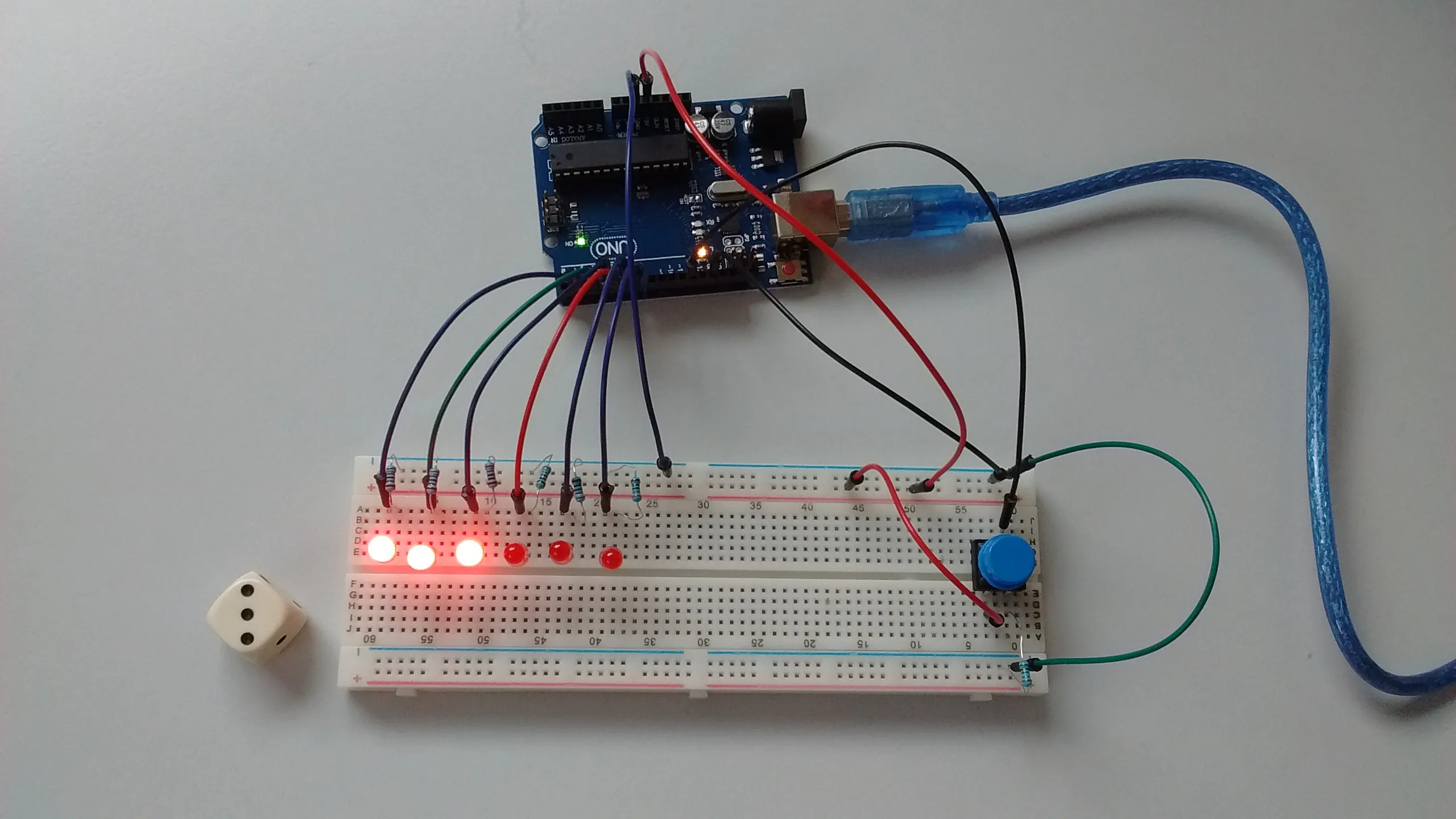# LED Dice

Learn how to simulate throwing a dice with the Arduino and 6 LEDs!

BeginnerShowcase (no instructions)39,714## Things used in this project

### Hardware componentsArduino UNO & Genuino UNO
×1LED (generic)
×6SparkFun Pushbutton switch 12mm
×1Jumper wires (generic)
×1Resistor 1k ohm
×1Resistor 221 ohm
×6

## Schematics

### Circuit schematic

This is the schematic circuit made with Fritzing for the dice prototype.

The circuit:
* 6 LEDs attached to consecutive digital pins (with 220 Ohm resistors)
* Button switch connected to digital pin (see circuit on https://www.arduino.cc/en/Tutorial/Button)### Circuit photo

This is the real-world circuit.## Code

### Dice

Arduino
With this code you can simulate throwing a dice, the number of LEDs lighting up is the number on the thrown dice.
```/*
Arduino Dice :)

This example shows how to simulate throwing a dice with 6 LEDs.

The circuit:
* 6 LEDs attached to consecutive digital pins (with 220 Ohm resistors)
* Button switch connected to digital pin (see circuit on https://www.arduino.cc/en/Tutorial/Button)

Created 5 Jan 2017
By Esther van der Stappen

This example code is in the public domain.

*/

// set to 1 if we're debugging
#define DEBUG 0

// 6 consecutive digital pins for the LEDs
int first = 2;
int second = 3;
int third = 4;
int fourth = 5;
int fifth = 6;
int sixth = 7;

// pin for the button switch
int button = 12;
// value to check state of button switch
int pressed = 0;

void setup() {
// set all LED pins to OUTPUT
for (int i=first; i<=sixth; i++) {
pinMode(i, OUTPUT);
}
// set buttin pin to INPUT
pinMode(button, INPUT);

// initialize random seed by noise from analog pin 0 (should be unconnected)

// if we're debugging, connect to serial
#ifdef DEBUG
Serial.begin(9600);
#endif

}

void buildUpTension() {
// light LEDs from left to right and back to build up tension
// while waiting for the dice to be thrown
// left to right
for (int i=first; i<=sixth; i++) {
if (i!=first) {
digitalWrite(i-1, LOW);
}
digitalWrite(i, HIGH);
delay(100);
}
// right to left
for (int i=sixth; i>=first; i--) {
if (i!=sixth) {
digitalWrite(i+1, LOW);
}
digitalWrite(i, HIGH);
delay(100);
}
}

void showNumber(int number) {
digitalWrite(first, HIGH);
if (number >= 2) {
digitalWrite(second, HIGH);
}
if (number >= 3) {
digitalWrite(third, HIGH);
}
if (number >= 4) {
digitalWrite(fourth, HIGH);
}
if (number >= 5) {
digitalWrite(fifth, HIGH);
}
if (number == 6) {
digitalWrite(sixth, HIGH);
}
}

int throwDice() {
// get a random number in the range [1,6]
int randNumber = random(1,7);

#ifdef DEBUG
Serial.println(randNumber);
#endif

return randNumber;
}

void setAllLEDs(int value) {
for (int i=first; i<=sixth; i++) {
digitalWrite(i, value);
}
}

void loop() {
// if button is pressed - throw the dice

if (pressed == HIGH) {
// remove previous number
setAllLEDs(LOW);

buildUpTension();
int thrownNumber = throwDice();
showNumber(thrownNumber);
}

}
```

## Credits

### EvdS

1 project • 13 followers